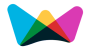#Online,Easy and Quick Business Loan Calculation#Business Loan Calculator

Instantly calculates your business loan EMI and total loan repayment for different loan periods.

Months
Repayment Term Calculation
1 to 5 Years   6 to 10 Years   11 to 15 YearsBusiness Loan calculator is an EMI calculator which helps you calculate your monthly repayments.

(All you have to do is, Just enter the loan amount, term of loan (in months) and the annual rate of interest, and you are done.)

The Business loan calculator uses the following formulas:

Monthly Repayments = ( P × r × (1+r)n ) / ((1+r)n - 1)

Amount Repayable = Monthly Payment × n

Where,

Monthly Repayments or EMI = Equated Monthly Installment

r = Interest rate / 1200

P = Loan Amount

n = Period

###More Calculators ►

Weekly Interest Calculator

Short Term Interest Calculator

Savings Interest Calculator